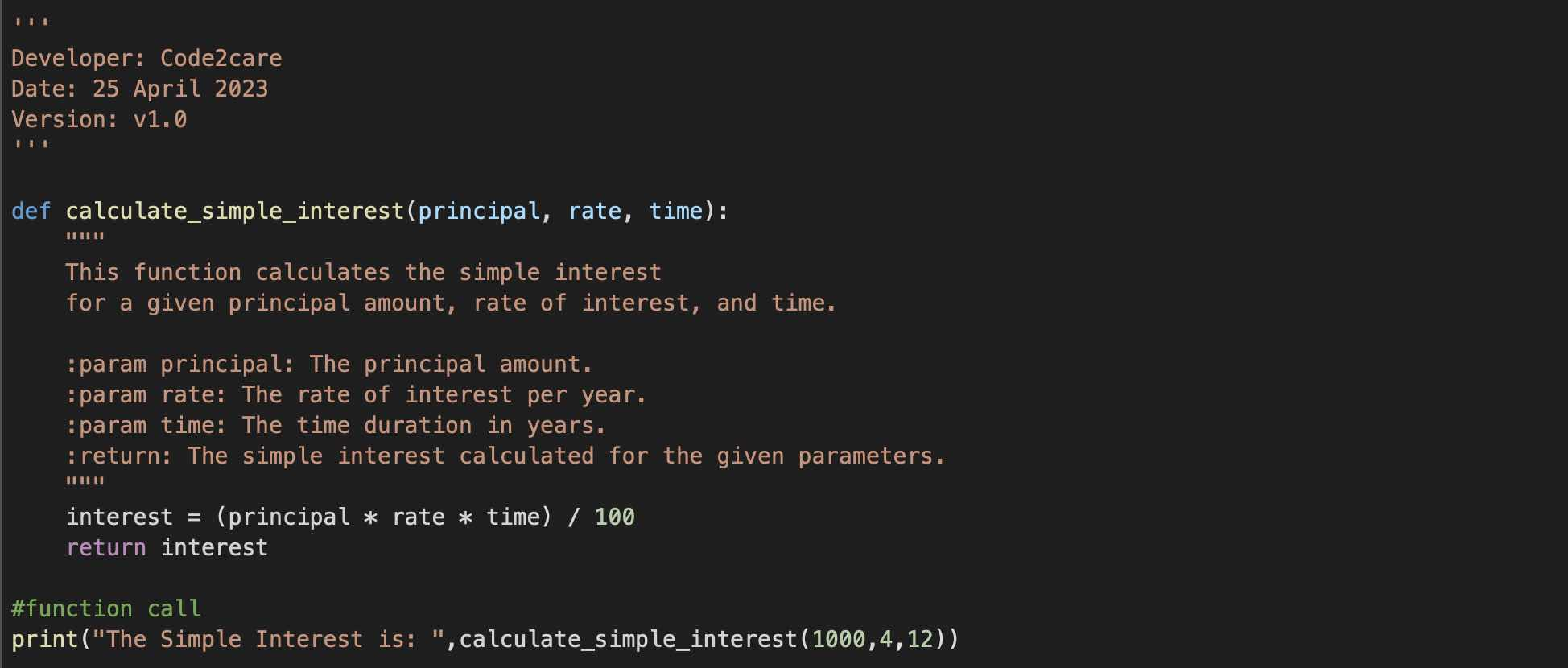There are two ways in which you can comment out multiple lines in Python Programming,

1. Multi-Line Comment: using triple single quotes: '''
2. Docstring: using triple double quotes: """

## Examples:

• Using triple single quotes at the start and end of the command line.
``````'''
This is a multi-line comment
using triple single quotes
that can span multiple lines.
'''

# This is a single-line comment

def my_function():
...
...
...
``````
• Using triple double quotes at the start and end of the command line.
``````def my_func():
"""
This is a multi-line comment
using triple single quotes
that can span multiple lines.

This is called the function-level docstring.
It is used to document the purpose and behavior of a function.
"""
...
...
...
``````

## Real World Example:

``````'''
Developer: Code2care
Date: 25 April 2023
Version: v1.0
'''

def calculate_simple_interest(principal, rate, time):
"""
This function calculates the simple interest
for a given principal amount, rate of interest, and time.

:param principal: The principal amount.
:param rate: The rate of interest per year.
:param time: The time duration in years.
:return: The simple interest calculated for the given parameters.
"""
interest = (principal * rate * time) / 100
return interest

#function call
print("The Simple Interest is: ",calculate_simple_interest(1000,4,12))``````-

Have Questions? Post them here!